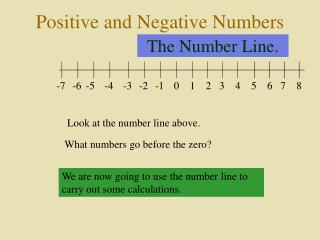DownloadDownload PresentationThe Number Line.

# The Number Line.

Télécharger la présentation## The Number Line.

- - - - - - - - - - - - - - - - - - - - - - - - - - - E N D - - - - - - - - - - - - - - - - - - - - - - - - - - -
##### Presentation Transcript

1. Positive and Negative Numbers The Number Line. -7 -6 -5 -4 -3 -2 -1 0 1 2 3 4 5 6 7 8 Look at the number line above. What numbers go before the zero? We are now going to use the number line to carry out some calculations.

2. Subtract -7 -6 -5 -4 -3 -2 -1 0 1 2 3 4 5 6 7 8 Adding and Subtracting. We are going to use the number line above to calculate the sum below: 6 – 10 = (1) Start at the number 6 (2) To subtract go left from 6. (3) Move 10 left. (4) Now answer the question 6 – 10 = -4

3. Add -7 -6 -5 -4 -3 -2 -1 0 1 2 3 4 5 6 7 8 Adding & Subtracting Example. Now calculate: -3 + 7 = -3 (1) At what number do you start? (2) To add do you move left or right? right (3) How many squares do you move? 7 (4) The answer to the sum -3 + 7 = is? 4

4. -7 -6 -5 -4 -3 -2 -1 0 1 2 3 4 5 6 7 8 Adding & Subtracting Example. Calculate – 2 – 3 = At what number do you start? How many steps do you move? Now complete the sum : – 2 – 3 = -5

5. What Goes In The Box ? Complete the questions below yourself: (1) 6 – 8 = -2 (6) 7 – 12 = -5 (2) – 2 + 6 = 4 (7) – 7 + 12 = 5 (3) – 5 + 7 = 2 (8) – 6 + 6 = 0 (4) –4 + 8 = 4 (9) – 2 – 5 = -7 (10) – 2 + 7 – 8 = -3 (5) –1 – 6 = -7

6. What Goes In The Box ? 2 Complete these questions using a bigger number line if required: - 48 (1) 12 – 16 = -4 (6) 72 – 120 = (2) – 32 + 26 = -6 (7) – 34 + 52 = 18 (3) – 35 + 43 = 8 (8) – 36 + 26 = -10 (4) –14 +28 = 14 (9) – 42 – 57 = -99 -45 (10) – 29 + 73 – 89 = (5) –18 – 52 = -70

7. What goes In The Box ? 3 Work backwards here to work out the missing numbers. (1) – 6 + = 12 18 (2) + 10 = – 3 -13 (3) 12 – = – 22 34 (4) – 14 = – 25 -11 (5) 22 – = – 17 39